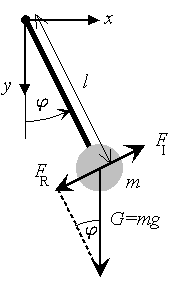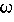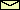# The Equation of Motionof an undamped and undriven pendulumAccording to Newton's laws the inertia force FI (i.e., mass times acceleration) has to be equal to the applied force. In our case, the applied force is the restoring force FR caused by gravity G. From the geometry of the problem (see figure), it is clear that

FR = -G sin= - mg  sin,

where m is the mass of the pendulum and g is the acceleration of gravity. Note that the negative sign is caused by the fact that the restoring force FR wants to bring the pendulum back to equilibrium (i.e.,= 0).

Next, we have to express the inertia force FI in terms of the angle. Assuming a rigid pendulum (i.e., its length l is fixed), the mass can move only on a circle with radius l. The position (i.e., the spatial coordinate) along this circle is given by l. Note that the angleis measured in radians (i.e., 180° corresponds to). The acceleration is therefore given by l d2/dt2. Thus, from Newton's law we get
ml d2/dt2 = -mg sin.

Dividing by ml and moving the term on the right-hand side to the left-hand side leads to the equation of motion of an undamped and undriven pendulum

 (1) d2/dt2 +02 sin= 0,

where

 (2)0 = (g/l)1/2.

• The equation of motion is a second-order differential equation (due to the second derivative of the angle). In order to get a unique solution, one needs two real numbers, e.g. the angle and the angular velocity at a specific time. Both variables define uniquely the state of the undriven pendulum.
• The equation of motion is nonlinear because the second term depends nonlinearly on the angle.
© 1998 Franz-Josef Elmer,Franz-Josef doht Elmer aht unibas doht ch, last modified Sunday, July 19, 1998.